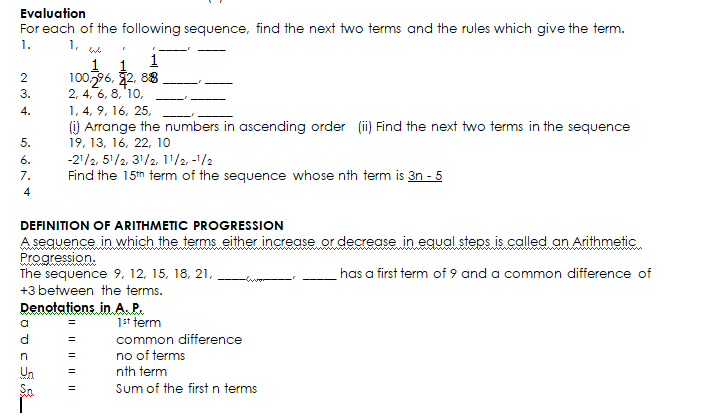# Arithmetic Progression

Welcome to class!

In today’s class, we will be talking about the arithmetic progression. Enjoy the class!

### Arithmetic ProgressionCONTENT

• Sequence
• Definition of Arithmetic Progression
• Denotations in Arithmetic progression
• Deriving formulae for the term of A. P.
• Sum of an arithmetic series

Find the next two terms in each of the following sets of number and in each case state the rule which gives the term.

(a)       1, 5, 9, 13, 17, 21, 25(any term +4 = next term)

(b)       2, 6, 18, 54, 162, 486, 1458 (any term x 3 = next term)

(c)        1, 9, 25, 49, 81, 121, 169, (sequence of consecutive odd no)

(d)       10, 9, 7, 4, 0, -5, -11, 18, -26, (starting from 10, subtract 1, 2, 3 from immediate no).

In each of the examples below, there is a rule which will give more terms in the list. A list like this is called a SEQUENCE in many cases; it can simply matter if a general term can be found for a sequence e.g.

1, 5, 9, 13, 17 can be expressed as

1, 5, 9, 13, 17 ……………. 4n – 3 where n = no of terms

Check: 5th term    = 4(5) -3

20 – 3 = 17

10th term = 4(10) – 3

40 – 3 = 37

Example 2

Find the 6th and 9th terms of the sequence whose nth term is

(a)       (2n + 1)

(b)       3 – 5n.

Solution

(a)       2n + 1

6th term            =   2(6) + 1   = 12 + 1 = 13

9th term            =   2 (9) + 1 = 18 + 1 = 19

(b)       3 – 5n

6th term            = 3 – 5 (6) = 3 – 30 = -27

9th term            = 3 – 5 (9) = 3 – 45 = –42###### GENERAL EVALUATION
1. An A. P. has 15 terms and a common difference of -3, find its first and last term if its sum is 120.
2. On the 1st of January, a student puts N10 in a box, on the 2nd she puts N20 in the box, on the 3rd she puts N30 and so on putting on the same no. of N10 notes as the day of the month. How much will be in the box if she keeps doing this till 16th January?
3. The salary scale for a clerical officer starts at N55, 200 per annum. A rise of N3, 600 is given at the end of each year, find the total amount of money earned in 12 years.
4. 4. Find the 7th  term and the nth term of the progression  27,9,3,…
5. If 8, x, y, – 4 are in A.P, find x and y.

In our next class, we will be talking about Geometric Progressions.  We hope you enjoyed the class.

Should you have any further question, feel free to ask in the comment section below and trust us to respond as soon as possible.

How Can We Make ClassNotesNG Better - CLICK to Tell Us💃